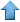Precision RecruitingSite Map
 [ Home ] [ Finance ] [ Web Audit ] [ Consulting ]

### Cryptography

Many cryptographic techniques rely on prime numbers. Their power comes from the virtual impossibility of factoring a product of two very large prime numbers.

Data Shaping Solutions offers an alternative to traditional cryptography. The detail of our algorithm are confidential. But you will find below interesting anecdotal results related to prime numbers.The function illustrated in the above graph has the following properties:

• It takes value between 0 and 1.
• It is equal to zero if and only if the argument is a prime number greater than 2.
• It is equal to one if and only if the argument is a composite number.
• It is a continuous function.
• The formula to compute this function is purely analytical, involving simple trigonometric functions and a finite number of power transformations. In particular, it does not involve prime numbers, nor moduli or divisors.
It belongs to a broad class of prime-generating functions investigated in our laboratory. Additional material can be found on our mathematical contest page.Data Mining • Machine Learning • Analytics • Quant • Statistics • Econometrics • Biostatistics • Web Analytics • Business Intelligence • Risk Management • Operations Research • AI • Predictive Modeling • Actuarial Sciences • Statistical Programming • Customer Insight • Data Modeling • Competitive Intelligence • Market Research • Information Retrieval • Computer Science • Retail Analytics • Healthcare Analytics • ROI Optimization • Design Of Experiments • Scoring Models • Six Sigma • SAS • Splus • SAP • ETL • SPSS • CRM • Cloud Computing • Electrical Engineering • Fraud Detection • Marketing Databases • Data Analysis • Decision Science • Text Mining